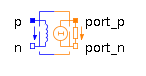Slew Rate Limiter - MapleSim Help

Slew Rate Limiter

Limits the slew rate of a signalDescription The Slew Rate Limiter block limits the slew rate of its output signal to the range $\left[\mathrm{Falling},\mathrm{Rising}\right]$. To ensure this for arbitrary inputs and in order to produce a differentiable output, the input is numerically differentiated with derivative time constant ${T}_{d}$. Smaller time constant ${T}_{d}$ means nearer ideal derivative. The user has to choose the derivative time constant according to the nature of the input signal. The Signal Size parameter assigns the dimension of the input and output connectors.Equations $\frac{{\mathrm{dy}}_{i}}{\mathrm{dt}}=\left\{\begin{array}{cc}\mathrm{smooth}\left(1,\mathrm{noEvent}\left(\mathrm{min}\left(\mathrm{Rising},\mathrm{max}\left(\mathrm{Falling},\frac{{u}_{i}-{y}_{i}}{{T}_{d}}\right)\right)\right)\right)& \mathrm{strict}\\ \mathrm{smooth}\left(1,\mathrm{min}\left(\mathrm{Rising},\mathrm{max}\left(\mathrm{Falling},\frac{{u}_{i}-{y}_{i}}{{T}_{d}}\right)\right)\right)& \mathrm{otherwise}\end{array}$Connections

 Name Description Modelica ID $u$ Real input vector u $y$ Real output vector yParametersGeneral Parameters

 Name Default Units Description Modelica ID Signal Size $1$ Dimension of input and output signals signalSize $\mathrm{Rising}$ $1$ $\frac{1}{s}$ Maximum rising slew rate Rising $\mathrm{Falling}$ $-\mathrm{Rising}$ $\frac{1}{s}$ Maximum falling slew rate Falling ${T}_{d}$ $0.001$ $s$ Time constant used to compute numerical derivative Td ${y}_{\mathrm{start}}$ $0$ Initial or guess value of output (=state) y_start Init type Steady state initialization (derivatives of states are zero) Type of initialization (SteadyState implies y = u) initTypeName Default Units Description Modelica ID strict $\mathrm{false}$ True (checked) ensures limits are strictly enforced via events strictModelica Standard Library The component described in this topic is from the Modelica Standard Library. To view the original documentation, which includes author and copyright information, click here.